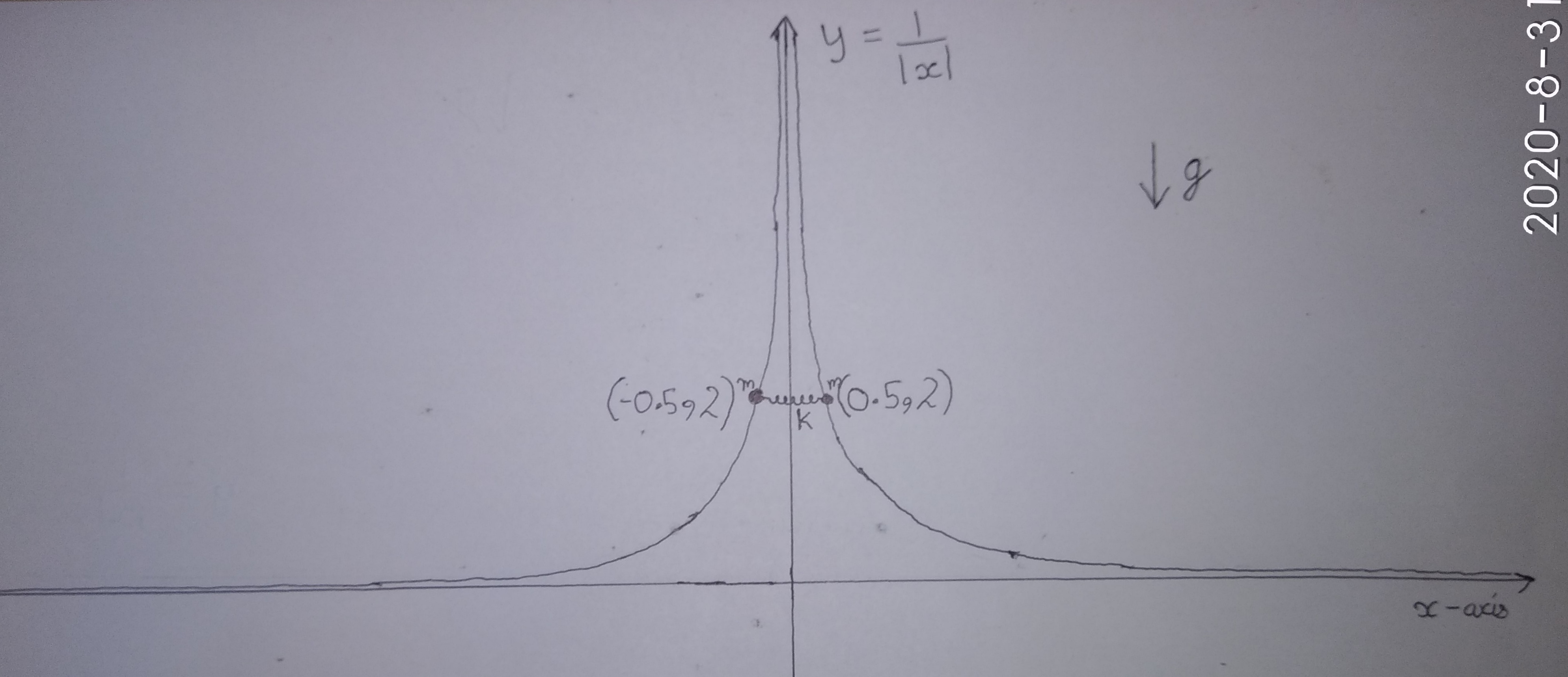# Graphical Mechanics(Part 4)Two rings each of mass $m$ are connected with a spring of stiffness $k$ and natural length $l$ as shown. Rings are constrained to move along $\text{wedge}$ shaped $\displaystyle y = \frac{1}{|x|}$. The system is released from $\text{rest}$. Find its Time Period $T$ in $\text{seconds}$.

Enter answer as $20T$.

# Details and Assumptions

• All surfaces are $\color{#3D99F6}{\text{smooth}}$

• Take acceleration due to gravity $\color{#3D99F6}{g = 10m/s^2}$

• Spring is $\color{#3D99F6}{\text{ideal}}$ and has natural length $\color{#3D99F6}{l = 2m}$

• Stiffness of spring $\color{#3D99F6}{k = 2mg \text{ N/m}}$

• Initially, rings are at $\color{#3D99F6}{(-0.5, 2)}$ and $\color{#3D99F6}{(0.5, 2)}$ respectively.

Inspiration Aniket Sanghi

Try similar problems by me

All of my problems are original

×